# TidyTuesday 2020-10-13

If you want to join the next CorrelAid TidyTuesday Meetup, make sure to sign up to our Newsletter or reach out to us on Twitter!

``````library(tidyverse)
library(tidytuesdayR)
library(ggplot2)
library(fontawesome) # for knitting, install with devtools::install_github("rstudio/fontawesome")
``````
``````##
``````

## A colorful dino!

By Christina

``````datasaurus_dozen <- tt\$datasaurus
ggplot(dplyr::filter(datasaurus_dozen, dataset=='dino'), aes(x, y, colour=-x)) +
geom_point(size=15, alpha=0.85, shape=16) + theme_bw() +
theme(legend.position = "none") +
scale_color_continuous(type = "viridis")
``````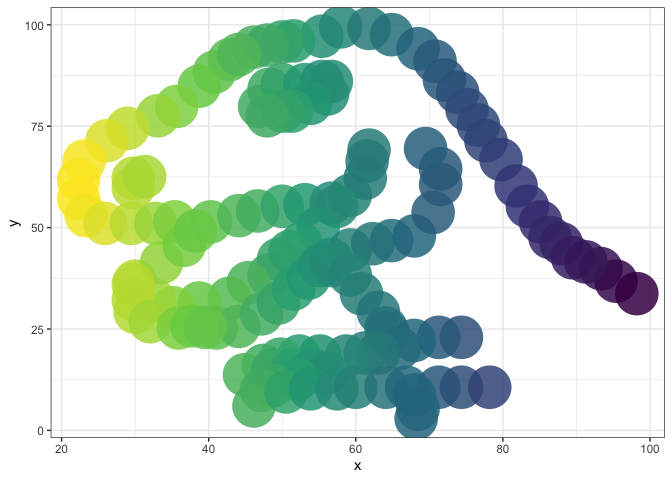## A colorful violin plot

By Lisa Reiber

``````ttd <- tt\$datasaurus
ttd %>%
ggplot(aes(x = x, y = y, fill = dataset, color = dataset)) +
geom_smooth(method = "lm", alpha = 0.1, color = "grey") +
geom_violin(alpha = 0.1) +
geom_point(alpha = 0.3) +
facet_wrap(~dataset) +
theme_light() +
theme(legend.position = "none",
panel.grid = element_blank()) +
labs(x = "", y = "") +
NULL
``````
``````## `geom_smooth()` using formula 'y ~ x'
``````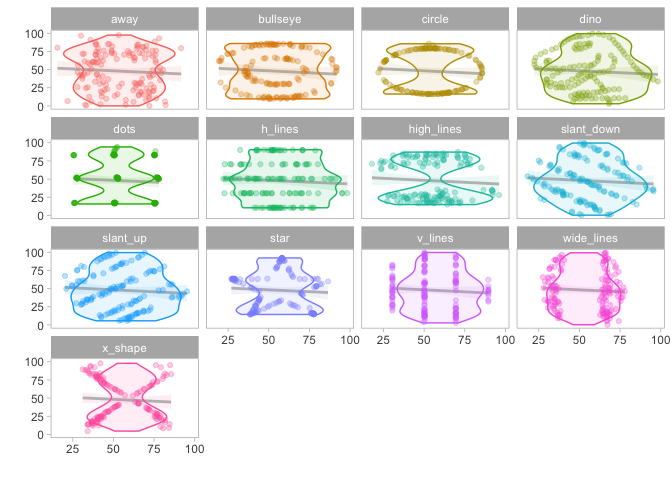## An animation!

By Frie Preu

``````library(gganimate)
library(cumstats) # for cumulative standard deviation
library(dplyr) # to use dplyr's cummean not cumstats'
``````
``````ds <- tt\$datasaurus
ds <- ds %>%
group_by(dataset) %>%
arrange(x, .by_group = TRUE) %>% # arrange by x to get cumulative values that we use for the animation
mutate(n = 1:n(), cum_mean_y = cummean(y), cum_var_y = cumvar(y)) %>%
mutate(cum_sd_y = sqrt(cum_var_y))

a <- ggplot(ds, aes(x = x, y = y))+
geom_point(color = "darkgrey", size = 0.5)+
geom_hline(aes(yintercept = cum_mean_y, color = dataset))+
geom_text(aes(label = round(cum_sd_y, 1), color = dataset), x = 85, y = 10, size = 3)+
theme_light()+
theme(legend.position = "none")+
labs(title = "Cumulative Mean and Standard Deviation of Y", caption = "Standard deviation in the bottom right corner of each plot.")+
facet_wrap(~dataset, ncol=4)

an <- a +
transition_time(n)+
# animate(an, renderer = gifski_renderer()) # commented out for knitting
# gganimate::anim_save("reveal_mean.gif") # commented out for knitting
``````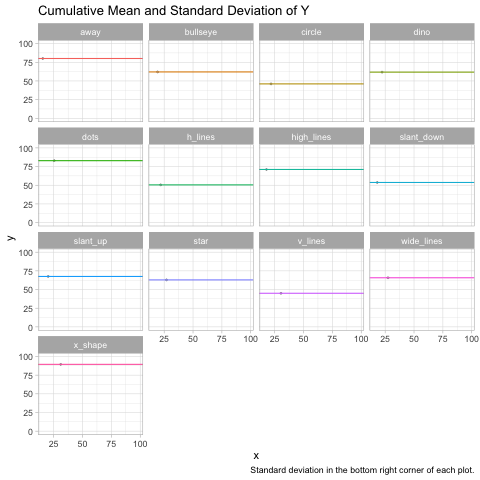late night #tidytuesday submission..i just couldn't wrap my head around the fact that indeed, those points really “end up” with the same mean and standard deviation. so i plotted it to convince myself. :) #rstats #ggplot pic.twitter.com/O2IoSPmCou

— Frie (@ameisen_strasse) October 13, 2020

Update: Long (see next contribution) did this amazing cyberpunk edit - for even better laser beams effects! Amazing theme, come through.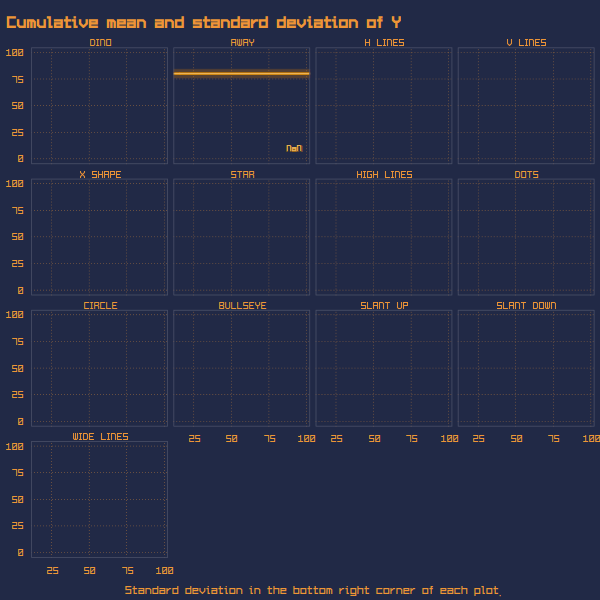## A joy plot - oh joy!

By Long Nguyen

Long created a helper function for the grid and also has his own ggplot theme which he shared here. This code here does not include the theme because it requires installing fonts etc.

``````library(tidyverse)
library(tidytext)
library(ggridges)
library(PerformanceAnalytics)
``````

#### Helper function `panel_grid`

``````panel_grid <- function(grid = "XY", on_top = FALSE) {
ret <- theme(panel.ontop = on_top)
if (grid == TRUE || is.character(grid)) {
if (on_top == TRUE)
grid_col <- "#ffffff"
else
grid_col <- "#cccccc"
ret <- ret + theme(panel.grid = element_line(colour = grid_col,
size = .2))
ret <- ret + theme(panel.grid.major = element_line(colour = grid_col,
size = .2))
ret <- ret + theme(panel.grid.major.x = element_line(colour = grid_col,
size = .2))
ret <- ret + theme(panel.grid.major.y = element_line(colour = grid_col,
size = .2))
ret <- ret + theme(panel.grid.minor = element_line(colour = grid_col,
size = .2))
ret <- ret + theme(panel.grid.minor.x = element_line(colour = grid_col,
size = .2))
ret <- ret + theme(panel.grid.minor.y = element_line(colour = grid_col,
size = .2))
if (is.character(grid)) {
if (!grepl("X", grid))
ret <- ret + theme(panel.grid.major.x = element_blank())
if (!grepl("Y", grid))
ret <- ret + theme(panel.grid.major.y = element_blank())
if (!grepl("x", grid))
ret <- ret + theme(panel.grid.minor.x = element_blank())
if (!grepl("y", grid))
ret <- ret + theme(panel.grid.minor.y = element_blank())
if (grid != "ticks") {
ret <- ret + theme(axis.ticks = element_blank())
ret <- ret + theme(axis.ticks.x = element_blank())
ret <- ret + theme(axis.ticks.y = element_blank())
} else {
ret <- ret + theme(axis.ticks = element_line(size = .2))
ret <- ret + theme(axis.ticks.x = element_line(size = .2))
ret <- ret + theme(axis.ticks.y = element_line(size = .2))
ret <- ret + theme(axis.ticks.length = grid::unit(4, "pt"))
}
}
} else {
ret <- theme(panel.ontop = FALSE)
ret <- ret + theme(panel.grid = element_blank())
ret <- ret + theme(panel.grid.major = element_blank())
ret <- ret + theme(panel.grid.major.x = element_blank())
ret <- ret + theme(panel.grid.major.y = element_blank())
ret <- ret + theme(panel.grid.minor = element_blank())
ret <- ret + theme(panel.grid.minor.x = element_blank())
ret <- ret + theme(panel.grid.minor.y = element_blank())
}
ret
}
``````

#### The plot

``````datasaurus <- tt\$datasaurus
p <- datasaurus %>%
pivot_longer(-dataset) %>%
ggplot(aes(value,
reorder_within(dataset, value, name, PerformanceAnalytics::kurtosis),
fill = stat(x))) +
geom_density_ridges_gradient(colour = "white", show.legend = FALSE,
scale = 3, rel_min_height = .01) +
scale_y_reordered() +
scale_fill_viridis_c(option = "A", direction = -1) +
facet_wrap(~name, scales = "free") +
labs(x = NULL, y = NULL, subtitle = "Ordered by kurtosis") +
panel_grid("Xx") +
theme(axis.text.y = element_text(vjust = 0))
# p # commented out for knitting , instead we include the themed version. see the gist for the theme
``````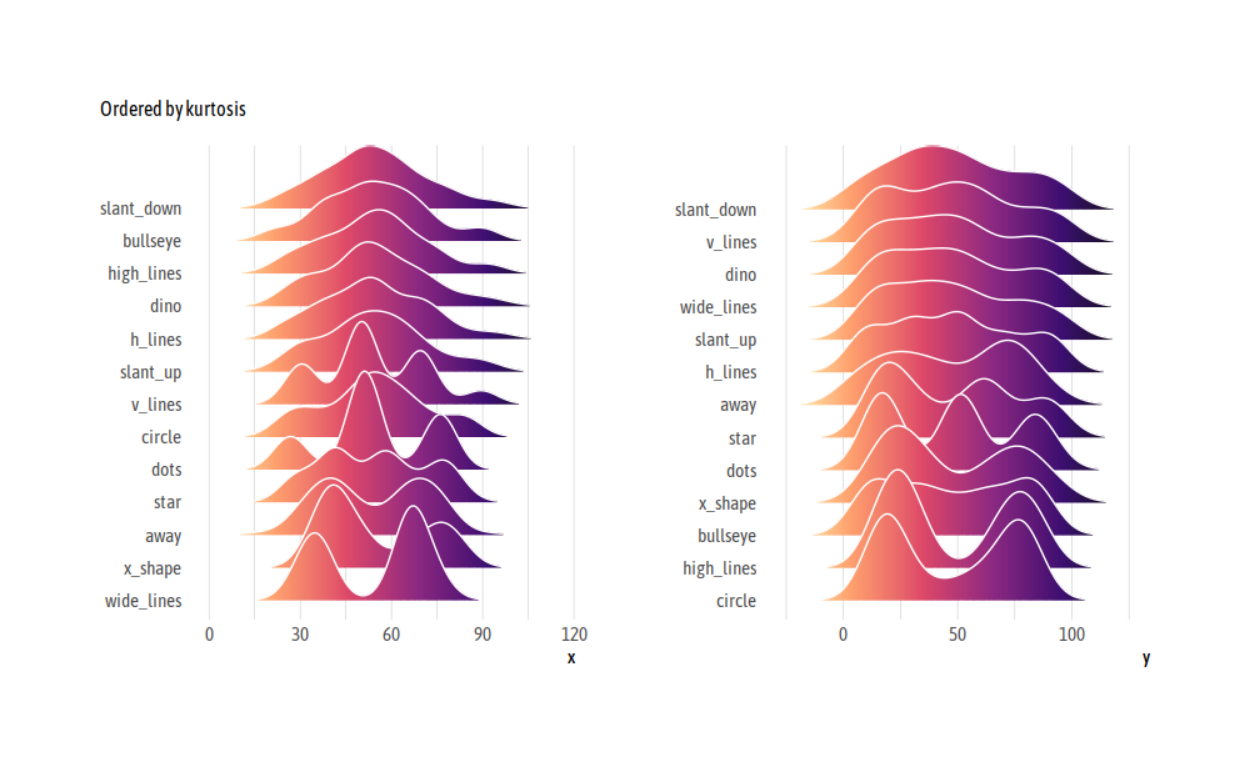## Resources and snippets from the Slack

1. If you want to check that it’s really true
``````ds %>%
group_by(dataset) %>%
summarize_all(list(mean = mean, sd = sd))
``````